Next: Paradoxes of lengths shortening Up: Kinematics of special relativity Previous: Relativity of simultaneity   Contents

# The Lorentz transformations

Let us make some comments concerning the Lorentz transformations. One of the approaches to deriving these transformations uses the light sphere, which is visible in different manner from two moving systems (the flash took place at the time of coincidence of the centers of systems). Or, what is actually the same, this approach uses the concept of interval (displaying the same sphere). The solution of the system of equations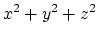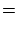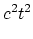(1.3)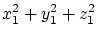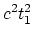(1.4)

represents simply the intersection of two surfaces and nothing more (Fig. 1.13).Under the condition of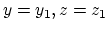these figures will be the surfaces of a sphere and of an ellipsoid of rotation with the distance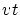between the centers of the figures. However, this is actually the other problem - the problem on two flashes: it is possible to find the centers of the given flashes for any time instant, i.e. to solve the reverse problem.

In the other approach to deriving the Lorentz transformations such a transformation is sought, which transfers equation (1.3) into equation (1.4). Obviously, for four variables such a transformation is not unique. First, the separate equatingrepresents only one of possible hypotheses, as well as the requirement of linearity, mutual uniqueness, reversibility, etc. (An additional possibility of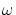-parametrization is described in Appendixes.) Second, any transformation of light surfaces does not determinate at all the transformation of volumes (in which the non-electromagnetic physical processes may occur). For example, the speed of sound does not depend on the motion of a source as well, but no global conclusions follow from this fact.

In any case, the Lorentz transformations in SRT physically describe two objects, rather than a single one. Otherwise it is easy to see a contradiction (Fig. 1.14).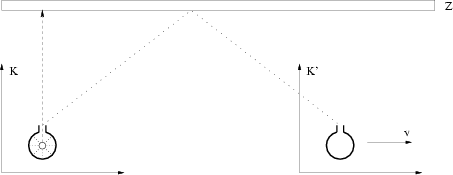Let a light flash occur. Let us separate, instead of a light sphere, one beam perpendicular to the mutual motion of systems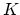and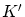(and let the remaining light energy be absorbed inside the system). Let us block the path of a beam by installing the long mirror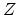at a great distance from sphere's center (along the line parallel to the line of mutual motion of systems). Then the observer situated at the center of systemwill record the reflected signal after some time. Let the signal be completely absorbed. However, the other observer moving together with systemwill catch a signal, also after some time, at the other point of space (let the signal be absorbed too). If we take a "continuum" of systems with different mutual velocities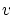, then the signal can be caught at any point of the straight line. Then where has the additional energy appeared from? May be this is SRT's perpetuum mobile of the first kind?

Note that if some mathematical equation is invariant relative the transformations of Lorentz type with some constant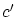, it means only that among particular solutions of this equation there exist "surfaces" of wave type which can propagate with the velocity. However, in this case even the given equation can have other particular solutions with other own invariant transformations, to say nothing of other mathematical equations, i.e. no overall mathematical conclusions do not follow from the fact of invariance. Only relativists try "to blow the big soap-bubble" from the particular phenomenon.Next: Paradoxes of lengths shortening Up: Kinematics of special relativity Previous: Relativity of simultaneity   Contents
Sergey N. Arteha